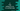# C program to find the ceiling of a number in a sorted array## C program to find the ceiling of a number in a sorted array:

In this C programming tutorial, we will learn how to find the ceiling value of a number in a sorted integer array. For our example, one sorted integer array is given. It will take one integer number as input from the user and print the ceil value for that number if it exists in that array.

ceiling value of a number is the smallest number that is greater than or equal to that number. For example, ceiling of 10 is 11 if it exists in the array.

### C program :

Let me write down the C program for this problem:

``````#include <stdio.h>

int getCeilingValue(int *arr, int size, int num)
{
if (num <= arr)
{
return arr;
}

if (num > arr[size - 1])
{
return -1;
}

int i;
for (i = 0; i < size; i++)
{
if (num == arr[i])
{
return arr[i];
}

if (arr[i] < num && arr[i + 1] > num)
{
return arr[i + 1];
}
}
}

int main()
{
int givenArray = {1, 3, 5, 7, 9, 11, 14, 18, 22, 33};
int givenNum;

printf("Enter a number to find ceil value : ");
scanf("%d", &givenNum);

int ceil = getCeilingValue(givenArray, 10, givenNum);

if (ceil == -1)
{
}
else
{
printf("Ceil value : %d\n", ceil);
}
return 0;
}``````

### Explanation:

• getCeilingValue method is used to get the ceiling value in an array for a number. Here, arr is the array, size is the size of the array and num is the number to check in the array.
• The first if statement checks if the given number is smaller than the first element of the array. If yes, we are returning the first array element.
• If the given number is greater than the last element of the array, return -1.
• The last for loop iterates through the numbers of the array one by one. If the number is equal to an array element, return that value.
• If the current element is greater than the current value and less than the next value, return the next value, which is just greater than num.
• The return value of this method is stored in the variable ceil. We are taking one number as input from the user and passing that value to this method. This method returns -1 if no ceil value is found. We are checking its value and based on that, we are printing one message.

### Sample output:

``````Enter a number to find ceil value : 15
Ceil value : 18

Enter a number to find ceil value : 13
Ceil value : 14

Enter a number to find ceil value : 14
Ceil value : 14

Enter a number to find ceil value : 100